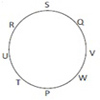# SAP Reasoning Question

DIRECTIONS for questions 1-2 : Solve the following question and mark the best possible option.
1. If SCIENTIST is coded as QAGCLRGQR then what will be the code for GENIUS?
1. ELCGSQ
2. ECLGRQ
3. ECLHSQ
4. ECLQSQ
5. ECLGSQ
Each letter is coded with a letter 2 behind it in the English alphabet.
S-2 = Q
C-2 = A.
Therefore code for GENIUS is ECLGSQ
2. If ROMAN is written as OBNPS then what will be the code for SUCCESS?
1. TVDDFTT
2. TTFEEVT
3. TTFDCVT
4. TTFDDVT
5. TTEDDVT
Reverse the word and write the respective next letter as the code for each letter.
Reverse of ROMAN is NAMOR , Now
N+1=O
A+1=B
Following same criteria for SUCCESS - SSECCUS
S+1=T
S+1=T
E+!=F
C+1=D
C+1=D
U+1=V
S+1=T
Therefore code is TTFDDVT
DIRECTIONS for the question 3 to 5: Solve the following question and mark the best possible option.
‘A$B’ means ‘A is smaller than B’ ‘A#B’ means ‘A is neither greater than nor smaller than B’ ‘A%B’ means ‘A is greater than B’ Now in each of the following questions assuming the given statements to be true, find which of the two conclusions I and II given below them is/are definitely true? 1.if only Conclusion I is true 2. if only Conclusion II is true 3. if either Conclusion I or II is true 4. if neither Conclusion I nor II is true 5. if both Conclusions I and II are true 1. Statements: X$Y, Y%Z, Z#L
Conclusions: I. L$X II. L%X 1. 1 2. 2 3. 3 4. 4 5. 5 Answer: Option D. X$Y means X is smaller than Y
Y%Z means Y is greater than Z
Z#L means Z is equal to L
Therefore, X < Y > Z = L
Conclusions :
I. L$X means L < X : Not True II. L%X means L>X : Not True 2. Statements: P$Q, Q#R, R%S
Conclusions: I. R$P II. Q%S 1. 1 2. 2 3. 3 4. 4 5. 5 Answer: Option B. P$Q means P is smaller than Q
Q#R means Q is equal to R
R%S means R is greater than S
Therefore,
P < Q = R > S
Conclusions :
I. R$P means R < P: Not True II. Q%S means Q > S : True 3. Statements: J$K, K#L, L%M
Conclusions: I. L$J II. K%M 1. 1 2. 2 3. 3 4. 4 5. 5 Answer: Option B. J$K means J is smaller than K
K#L means K is equal to L
L%M means L is greater than M
Therefore, J < K = L > M
Conclusions :
I. L$J means L < J : Not True II. K%M means K > M : True DIRECTIONS for the question 6 & 7 : Solve the following question and mark the best possible option. ‘P$Q’ means ‘P is either smaller than or equal to Q‘
‘P@Q’ means ‘P is either greater than or equal to Q’
‘P#Q’ means ‘P is neither greater than nor smaller than Q’
‘P%Q’ means ‘P is smaller than Q’
‘P! Q’ means ‘P is greater than Q
Now in each of the following questions assuming the given statements to be true, find which of the two conclusions I and II given below them is/are definitely true?
A)if only Conclusion I is true
B)if only Conclusion II is true
C)if either Conclusion I or II is true
D)if neither Conclusion I nor II is true
E)if both Conclusion I and II are true
4. Statements: A$B, B@C Conclusions: I. C%A II. A%C 1. 1 2. 2 3. 3 4. 4 5. 5 Answer: Option D. A$B means A is smaller than or equal to B
B@C means B is greater than or equal to C
Therefore, A <= B >= C
Conclusions :
I. C%A means C < A : Not True
II. A%C means A < C : Not True
5. Statements: M$L, N%M Conclusions: I. N$L II. N%L
1. 1
2. 2
3. 3
4. 4
5. 5
M$L means M is smaller than or equal to L N%M means N is smaller than M Therefore, N < M <= L Conclusions : I. N$L means N ≤ L : Not True
II. N%L means N < L : True
DIRECTIONS for the question 8 to 10 : Read the information given below and answer the question that follows.
P, Q, R, S, T, U, V and W are sitting around a circle facing at the center. S is second to the left of U and third to the right of W. P is second to the right of U and an immediate neighbour of W. R is second to the right of Q and U is third to the right of Q. V is not an immediate neighbour of U.
6. How many of them are there between W and R?
1. 2
2. 3
3. 2 or 3
5. None of these
According to the given information we can make the following diagramThere are 3 persons are between W and R.
7. Who is to the immediate left of P?
1. W
2. T
3. V
4. P
5. None of these